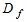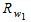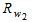# EFFECT OF WATER TABLE ON SAFE BEARING CAPACITY OF SOIL

The position of ground water has a significant effect on the bearing capacity of soil. Presence of water table at a depth less than the width of the foundation from the foundation bottom will reduce the bearing capacity of the soil.

The bearing capacity equation incorporating the ground water table correction factors is given below.Where= Ultimate bearing capacity of soil inc = Cohesion of soil inNc, Nq, N? are Therzaghi’s bearing capacity constants.= depth of foundation in meters

B = Width of the foundation in metersandare water table correction factors

The water table correction factors can be obtained from the equations given below.

1. When the water table is below the base of foundation at a distance ‘b’ the correctionis given by the following equation;

when b =0,= 0.5

2. When water table further rises above base of foundation, correction factorcomes in to action, which is given by the following equation.when a =,= 0.5Fig 1: Showing the influence of water table below foundation

The use of these equations is explained with the help of the Fig 1.

First let us begin with the correction factorWhen water table is at a depth greater than or equals to the width of foundation, from the foundation bottom, the correction factoris 1. i.e. there is no effect on the safe bearing capacity.

Let us assume water table started rising then the effect ofcomes in to action. The correction factor will be less than 1. When the water table reaches the bottom of foundation, i.e, when b = 0,= 0.5.

Now let us assume water table further raises, above the depth of foundation. When the depth of water table is just touching the bottom of foundation, a = 0. This means= 1.0. On further rising, when the water table reaches the ground level, Rw1 becomes 0.5.

Hence, the assessment of ground water level is an important aspect in any site investigation.